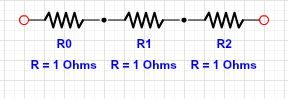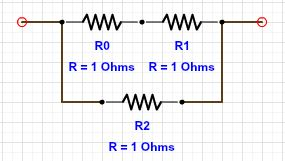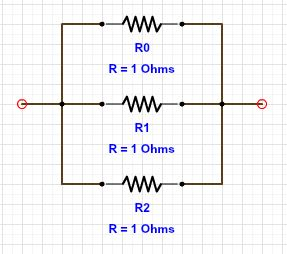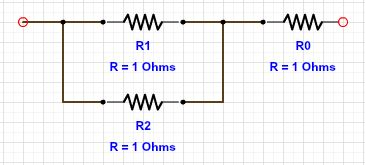# Equivalence of Resistors

Circuit: 1Circuit: 2Circuit: 3Circuit: 4In all the above circuits, there are three resistors each of resistance $1 \Omega$. Let $R_{eq_1}$, $R_{eq_2}$, $R_{eq_3}$ and $R_{eq_4}$ be the equivalent resistances of the circuit $1$, $2$, $3$ and $4$ respectively.

What is the correct statement about their equivalent resistances?

×

Problem Loading...

Note Loading...

Set Loading...